# 通过这14点，让你快速入门C语言（3）

## 10. 操作符

### 10.1 算术操作符

+
-
*
/
%

#include <stdio.h>

int main()
{
printf("%d\n", 8 / 2);//4
printf("%d\n", 7 / 2);//3(7 / 2=3...1)
printf("%lf\n", 7.0 / 2);//3.5   /两端至少有一个数是小数时除出来才是小数
printf("%.2lf\n", 7.0 / 2);//3.50
return 0;
}


#include <stdio.h>

int main()
{
printf("%d\n", 8 % 2);//0(8/2=4...0)      % - 取模/取余
return 0;
}


### 10.2 移位操作符

>>右移位操作符
<<左移位操作符
int main()
{
int a = 1;//int类型是4个字节 = 32bit   00000000000000000000000000000001
a >> 1;//00000000000000000000000000000001整体向右移一个二进制位
a << 1;//00000000000000000000000000000001整体向左移一个二进制位
return 0;
}


&按位与
|按位或
^按位异或

### 10.4 赋值操作符

=
+=
-=
*=
/=
&=
|=
^=
>>=
<<=
int main()
{
int a = 0;
a = 10;

a = a + 5;
a += 5;//这两种写法是一个效果

a = a - 3;
a -= 3;//这两种写法是一个效果

a *= 3;
a /= 3;

return 0;
}


### 10.5 单目操作符

!逻辑反操作
-负值
+正值
&取地址
sizeof操作数的类型长度（以字节为单位）
~对一个数的二进制按位取反
--前置、后置--
++前置、后置++
*间接访问操作符（解引用操作符）
（类型）强制类型转换

(i) "+"是一个操作符,"2+3"中2为左操作数，3为右操作数，因此+双目操作符，由此可知只有一个操作数的操作符就是单目操作符
(ii) C语言中0表示非0表示（非0：1、5、-1……）

//!叫做逻辑反操作，它只关注真假（把真变为假，把假变为真）
#include <stdio.h>

int main()
{
int a = 0;
scanf("%d", &a);//5   0          scanf函数中的&就是取地址

printf("%d\n", a);//5   0
printf("%d\n", !a);//0   1（假变为真时，语法规定输出的是1）

return 0;
}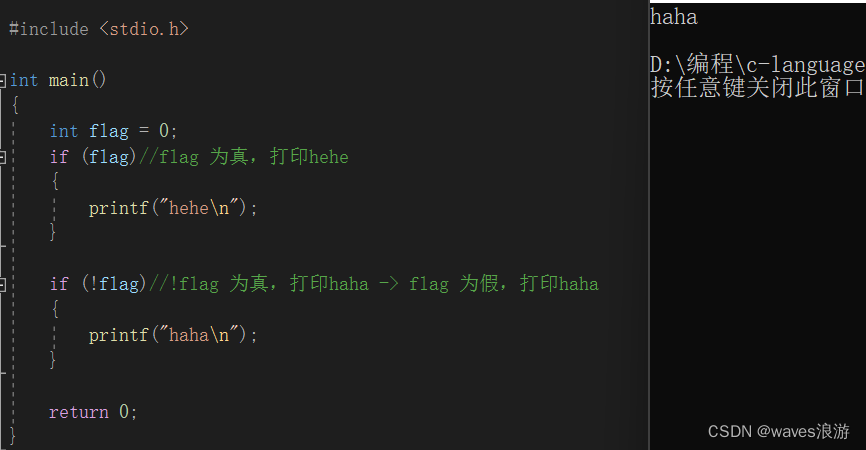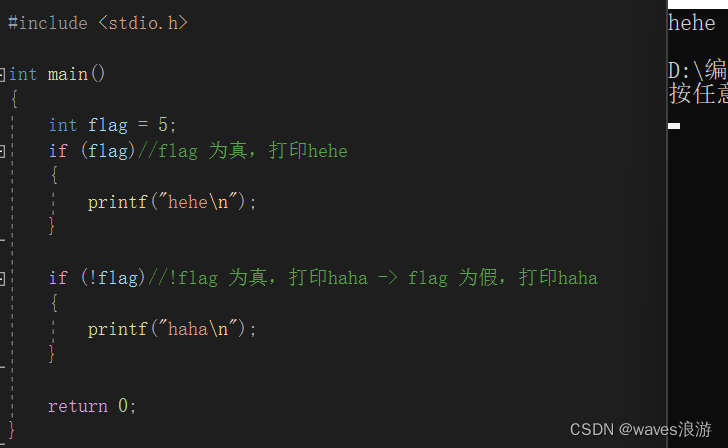#include <stdio.h>

int main()
{
int a = -10;
int b = -a;
printf("%d\n", b);//10

int c = +a;
printf("%d\n", c);//-10

return 0;
}


sizeof：

//sizeof是操作符,不是函数，计算的结果单位是字节
#include <stdio.h>

int main()
{
int a = 10;
printf("%d\n", sizeof(a));//4
printf("%d\n", sizeof a );//4    这里不加（）也可以，而函数必须加（），所以sizeof不是函数，是操作符！！！

printf("%d\n", sizeof(int));
printf("%d\n", sizeof int );//这样写是错误的

return 0;
}


#include <stdio.h>

int main()
{
int arr = { 0 };
printf("%d\n", sizeof(arr));//40，计算的是数组的总大小，单位是字节
printf("%d\n", sizeof(arr));//4
printf("%d\n", sizeof(arr) / sizeof(arr));//40 / 4 = 10

//因此数组中元素个数的计算如下
int sz = sizeof(arr) / sizeof(arr);

return 0;
}


#include <stdio.h>
#include <string.h>

int main()
{
char arr1[] = "abc";//a b c \0
char arr2[] = { 'a', 'b', 'c' };//a b c

printf("%d\n", strlen(arr1));//3
printf("%d\n", strlen(arr2));//随机值

printf("%d\n", sizeof(arr1));//4    sizeof只关注这个数据占据内存空间的大小，不关注内存中放的是什么
printf("%d\n", sizeof(arr2));//3

return 0;
}


#include <stdio.h>

int main()
{
int a = 10;
int b = ++a;//前置++，先++，后使用(a=a+1,b=a;)
printf("a=%d b=%d\n", a, b);//11  11

int b = a++;//后置++，先使用，后++(b=a,a=a+1;)
printf("a=%d b=%d\n", a, b);//11  10

int b = --a;//(a=a-1,b=a;)
printf("a=%d b=%d\n", a, b);//9  9

int b = a--;(b=a,a=a-1;)
printf("a=%d b=%d\n", a, b);//9  10

return 0;
}


#include <stdio.h>

int main()
{
int a = 1;
int b = (++a) + (++a) + (++a);
printf("b=%d\n", b);
return 0;
}



#include <stdio.h>

int main()
{
//int   double
int a = (int)3.14;
printf("%d\n", a);//3

return 0;
}


>大于
>=大于等于
<小于
<=小于等于
!=用于测试“不相等”
==用于测试“相等”

### 10.7 逻辑操作符

&&逻辑与（并且，只关注真假）
||逻辑或（或者，只关注真假）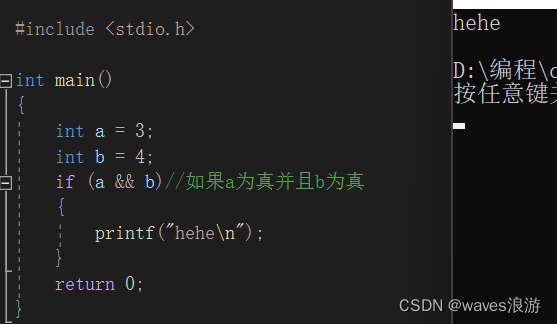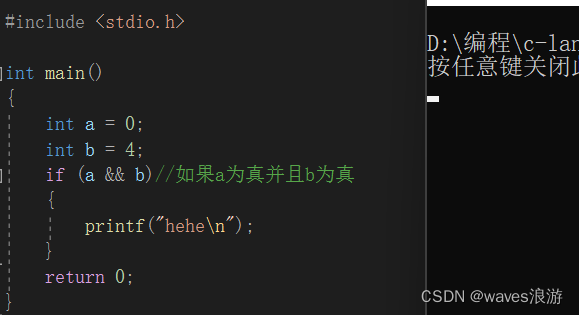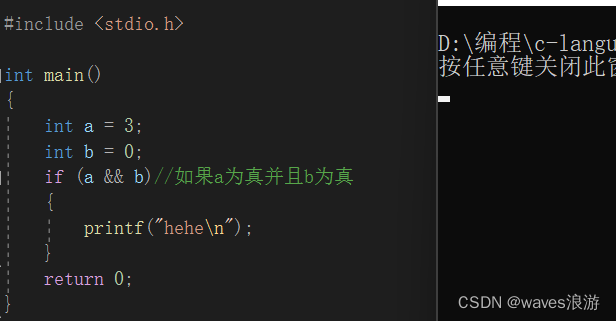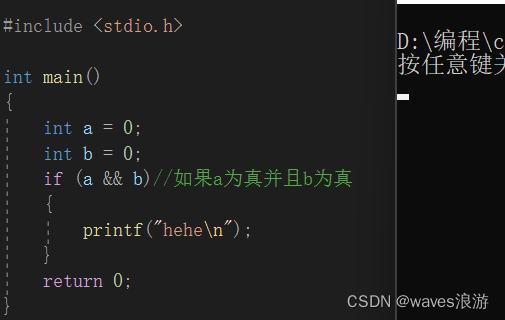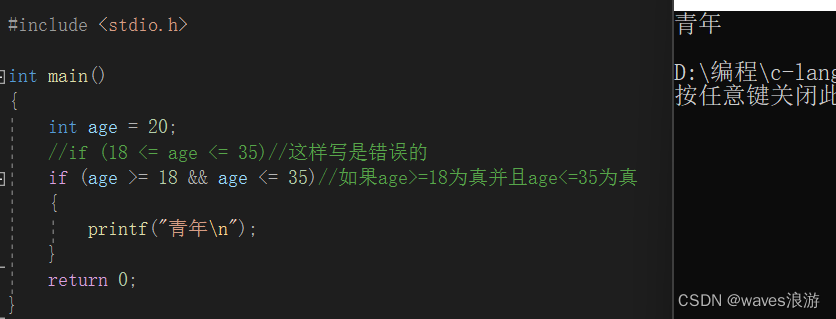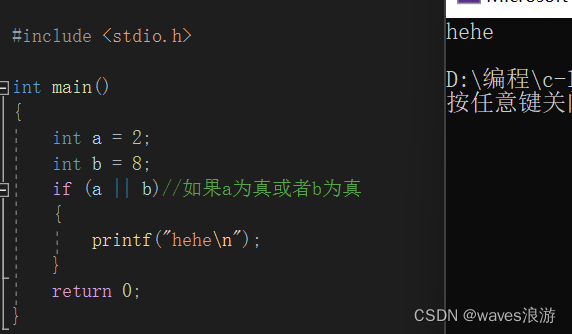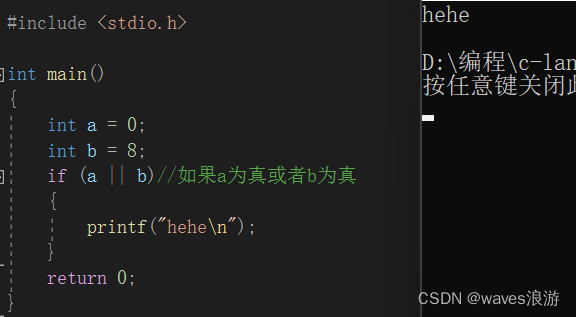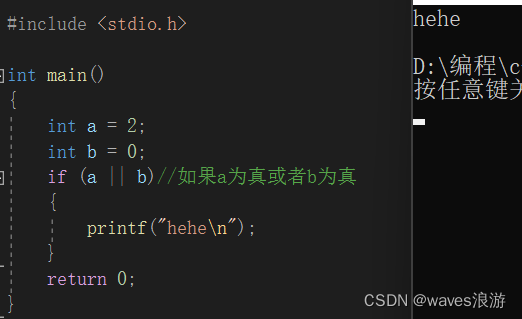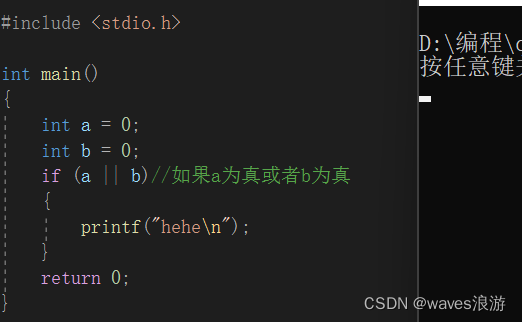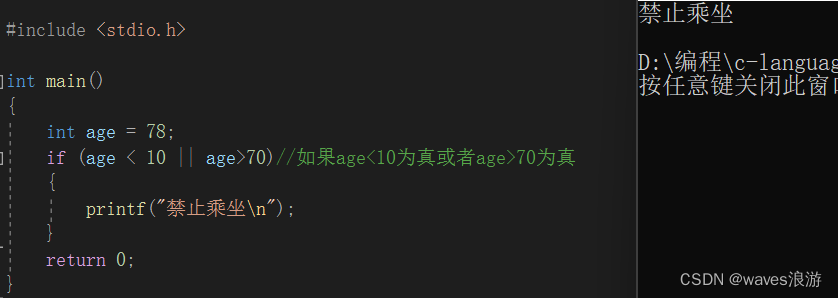### 10.8 条件操作符

exp1 ? exp2 : exp3
#include <stdio.h>

int main()
{
int a = 3;
int b = 7;
int m = 0;
m = (a > b ? a : b);//如果a>b，那么a，否则b（以下写法效果和它相同）
//if (a > b)
//	  m = a;
//else
//	  m = b;
printf("m=%d\n", m);//m=7
return 0;
}


exp1exp2exp3

### 10.9 逗号表达式

exp1, exp2, exp3, …expN
#include <stdio.h>

int main()
{
int a = 3;
int b = 0;
int c = 4;

int d = (a = b - 3, b = a + c, c = a - b, a = c + 3);
//a=-3      b=1        c=-4       a=-1
printf("%d\n", d);//-1
return 0;
}


### 10.10 下标引用、函数调用和结构成员

[ ]( ).
->

#include <stdio.h>

int main()
{
int arr = { 1,2,3,4,5,6,7,8,9,10 };
printf("%d\n", arr);//8;[]就是下标引用操作符,arr,7是[]的两个操作数

//2 + 3;2，3是+的两个操作数
return 0;
}


int Add(int x, int y)
{
return x + y;
}

int main()
{
int a = 10;
int b = 20;

return 0;
}


“.”、" ->"是结构体中使用的，后期介绍

## 11. 常见关键字

C语言提供了丰富的关键字，这些关键字都是语言本身预先设定好的，用户自己是不能创造关键字的。

break（停止）switchcharenum（枚举）extern（声明外部符号的）
continue（继续)caseshortstruct（结构体）register（寄存器关键字）
do whilebreakintunion（联合体/共用体）return（函数中返回）
fordefault（默认选项）longvolatile（这个关键字后面再讲）
whileif…else…long longauto（自动变量）
gotofloat
double
const
sizeof
static
signed（有符号的）
unsigned（无符号的）
typedef（类型重定义）
void（无类型/空类型，用于函数返回值、参数这些地方）

### 11.1 auto

int main()
{
auto int a = 10;//所有的局部变量都是自动变量，所以auto一般就省略不写。局部变量a进入它的作用域自动创建，出它的作用域自动销毁
return 0;
}


### 11.2 unsigned

int main()
{
unsigned int num = -10;//现阶段只要知道是怎么写的就可以了，具体输出后结果是什么以后会进行讲解
return 0;
}


### 11.3 typedef

typedef unsigned int unit;//给unsigned int重新取了一个名字叫做unit，unit = unsigned int

int main()
{
unsigned int num1 = 10;
unit num2 = 20;
return 0;
}


//数据结构

typedef struct Node
{
int data;
struct Node* next;
}Node;

//这一段代码的意思为给
//struct Node
//{
//	  int data;
//	  struct Node* next;
//}
//重新取了一个名字叫做Node

//现在只要大致能看懂就行，后期会深入讲解


### 11.4 register

int main()
{
register int num = 10;//register 起一个建议的作用，并不是写了register就一定会放到寄存器里，还是取决于编译器的，编译器如果发现这一变量被频繁大量使用，那么这个变量的值就可能被放到寄存器中，如果编译器觉得没必要把它放到寄存器中，那它就不会被放进去。即使不写register，现在的编译器只要觉得有必要把它放到寄存器中，编译器就会把它放到寄存器中
return 0;
}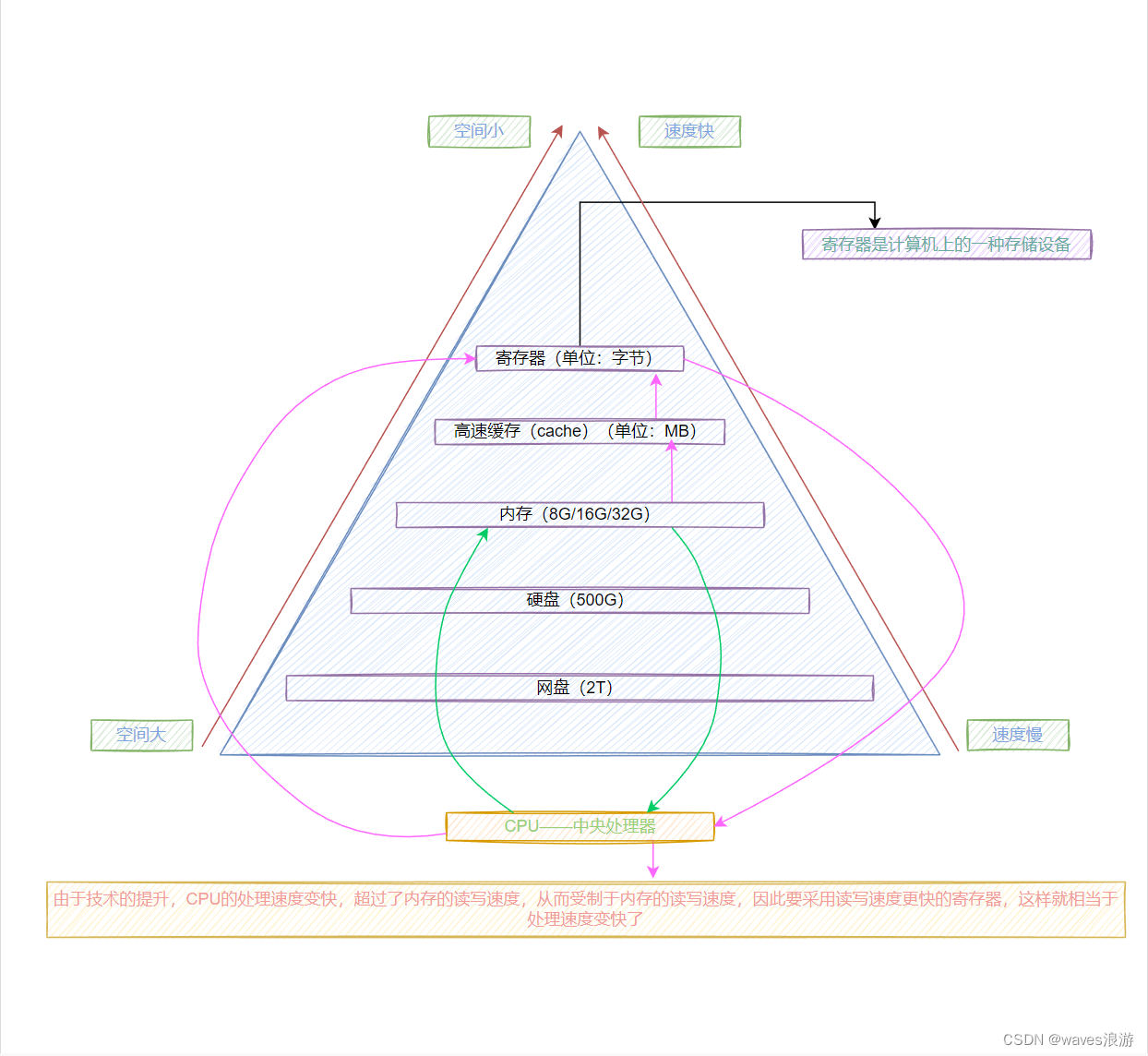### 11.5 static

• 修饰局部变量-称为静态局部变量
• 修饰全局变量-称为静态全局变量
• 修饰函数-称为静态函数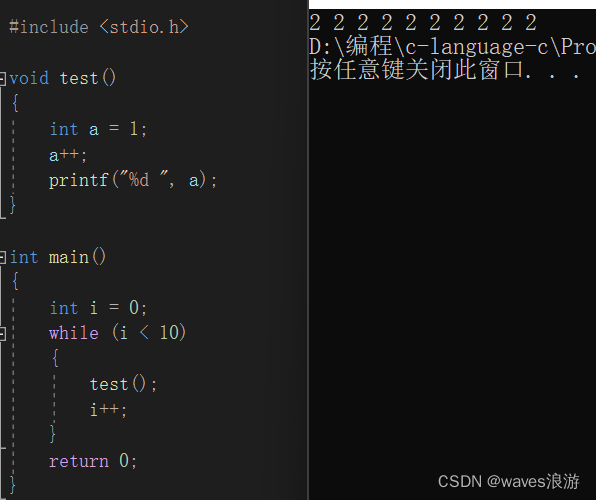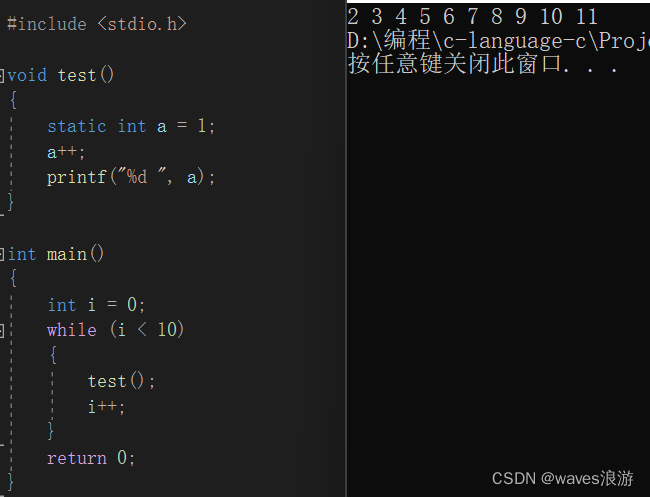static修饰局部变量代码运行过程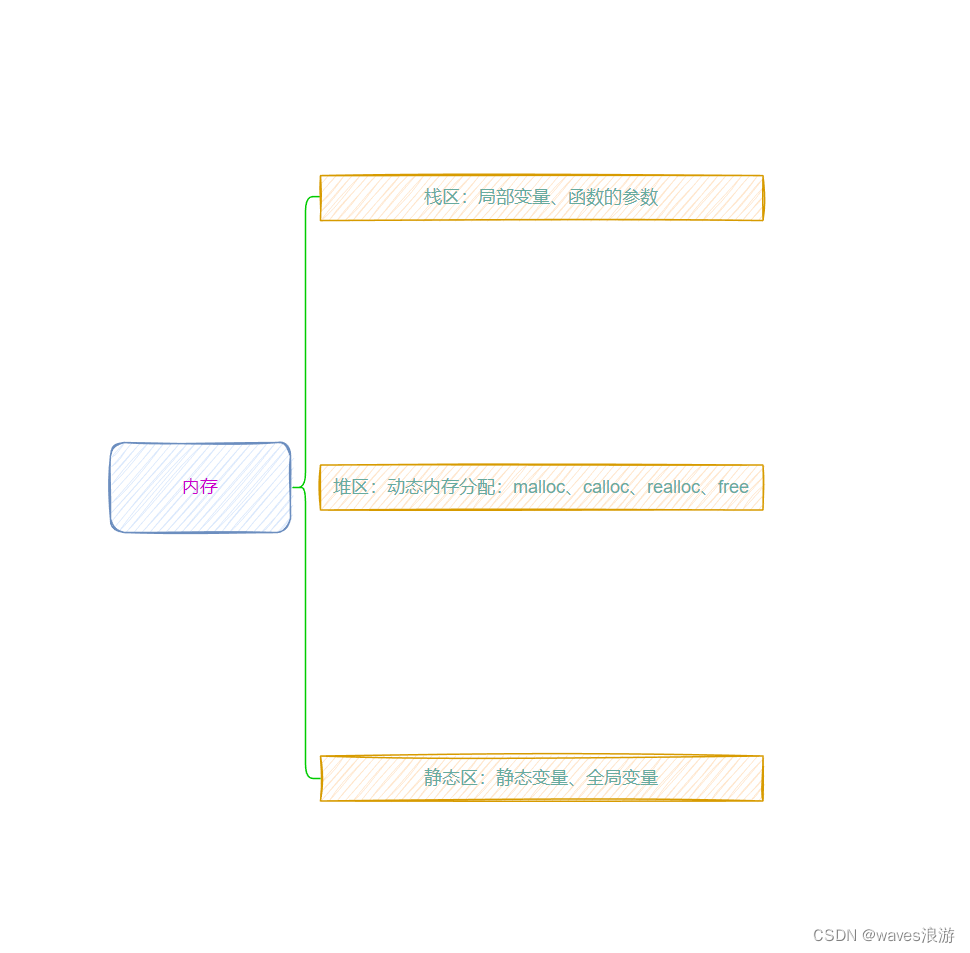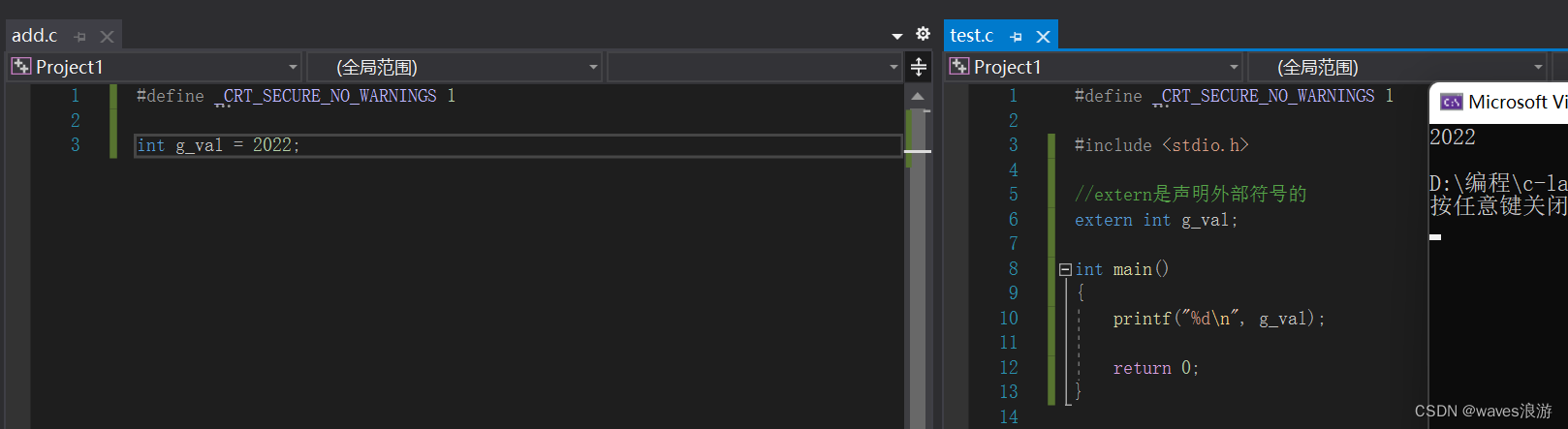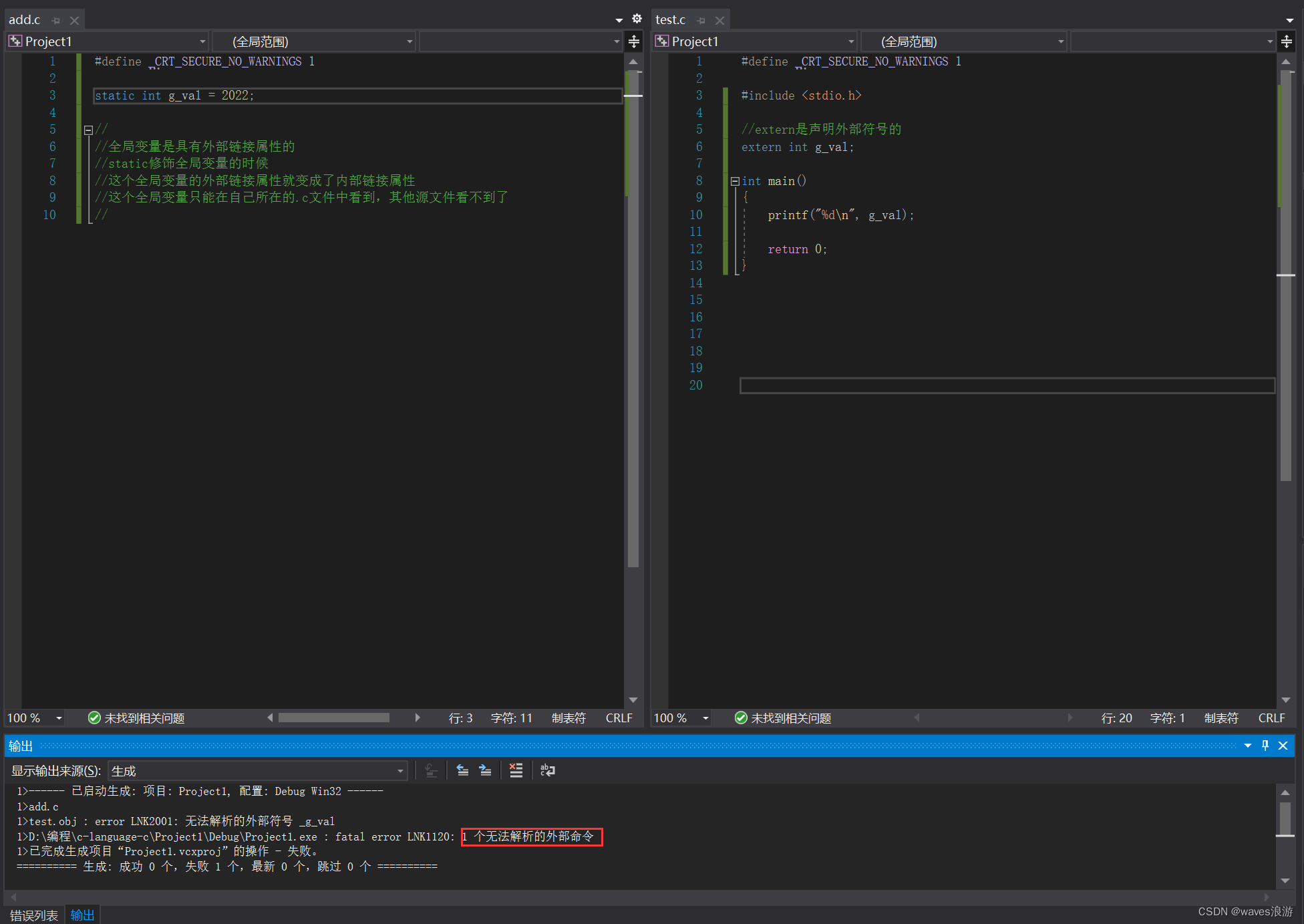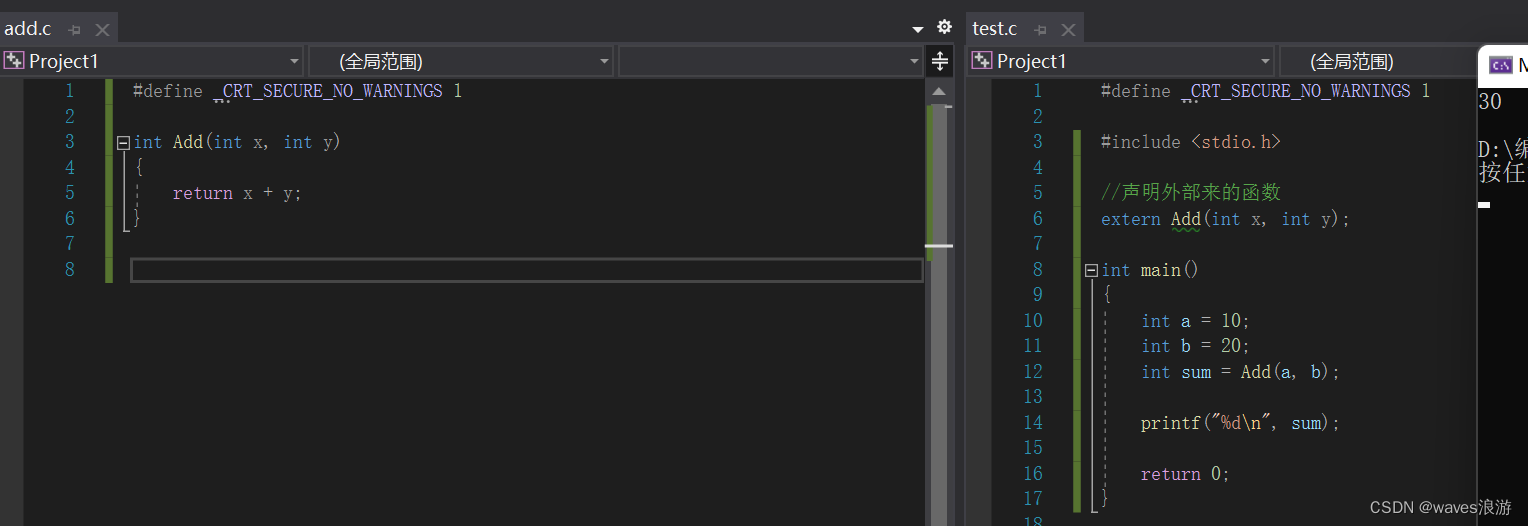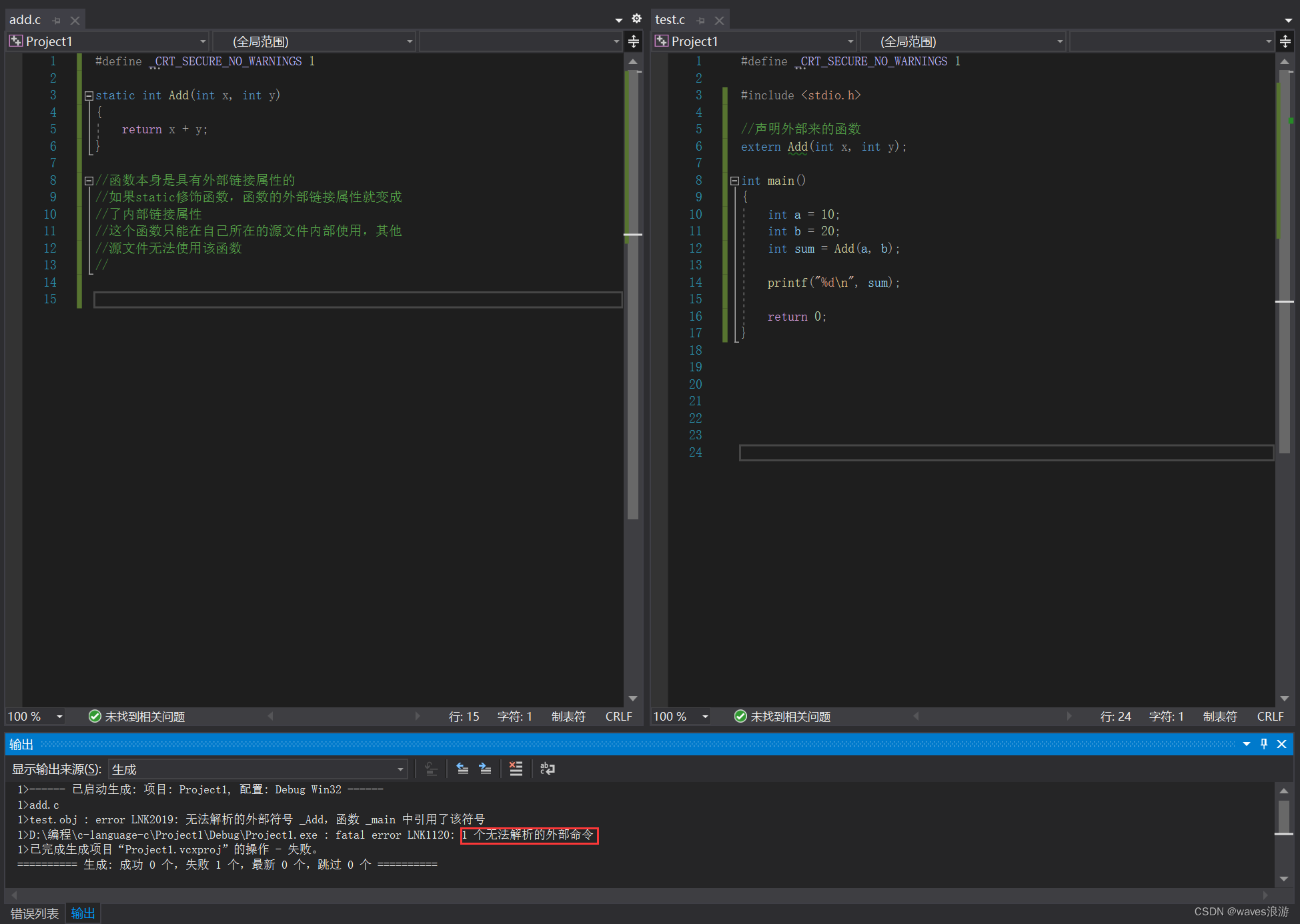# 附：

09-17
09-04
08-31
09-30
06-12
10-20
10-19
06-30
08-07
08-26
07-11
10-26
10-11
05-05

### “相关推荐”对你有帮助么？

•非常没帮助
•没帮助
•一般
•有帮助
•非常有帮助被折叠的  条评论 为什么被折叠?到【灌水乐园】发言waves浪游

¥2 ¥4 ¥6 ¥10 ¥20余额支付 (余额：-- )扫码支付获取中扫码支付点击重新获取扫码支付1.余额是钱包充值的虚拟货币，按照1:1的比例进行支付金额的抵扣。
2.余额无法直接购买下载，可以购买VIP、C币套餐、付费专栏及课程。余额充值